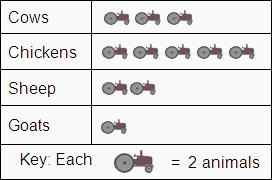Skill 6A
Pictograph
A pictograph is simply a way to represent quantities by using pictures.
Students must learn to look carefully at the "Key" which is at the bottom or side of each pictograph.  The Key is used to determine the amount represented by each picture.  Sometimes each picture represents amounts more than one.

Use the pictograph to answer each question.
Choose the correct answer from below the question.

Farm Animals

1. How many cows are on the farm?3 4 6 8 not sure
2. How many chickens are on the farm
 10 5 15 3 not sure
3. Which number sentence shows how many chickens and goats there are in all?
 A. 3 + 1 = 4 B. 5 + 1 = 5 C. 10 + 1 = 11 D. 10 + 2 = 12 E. not sure